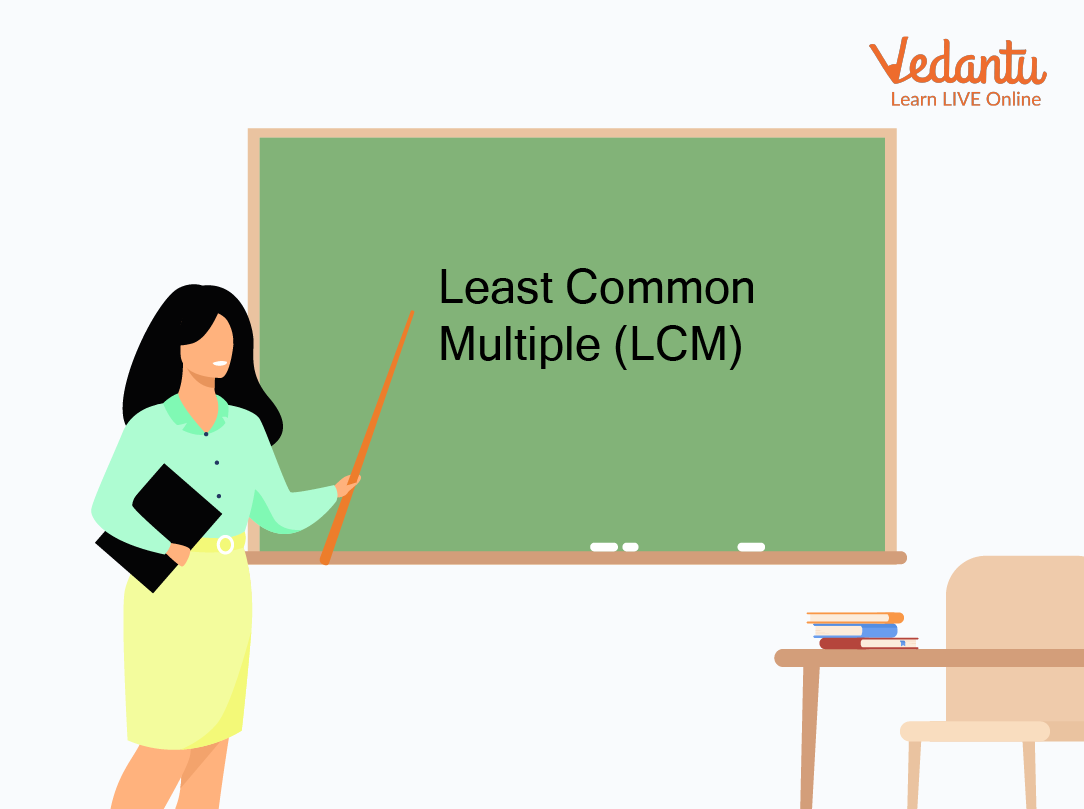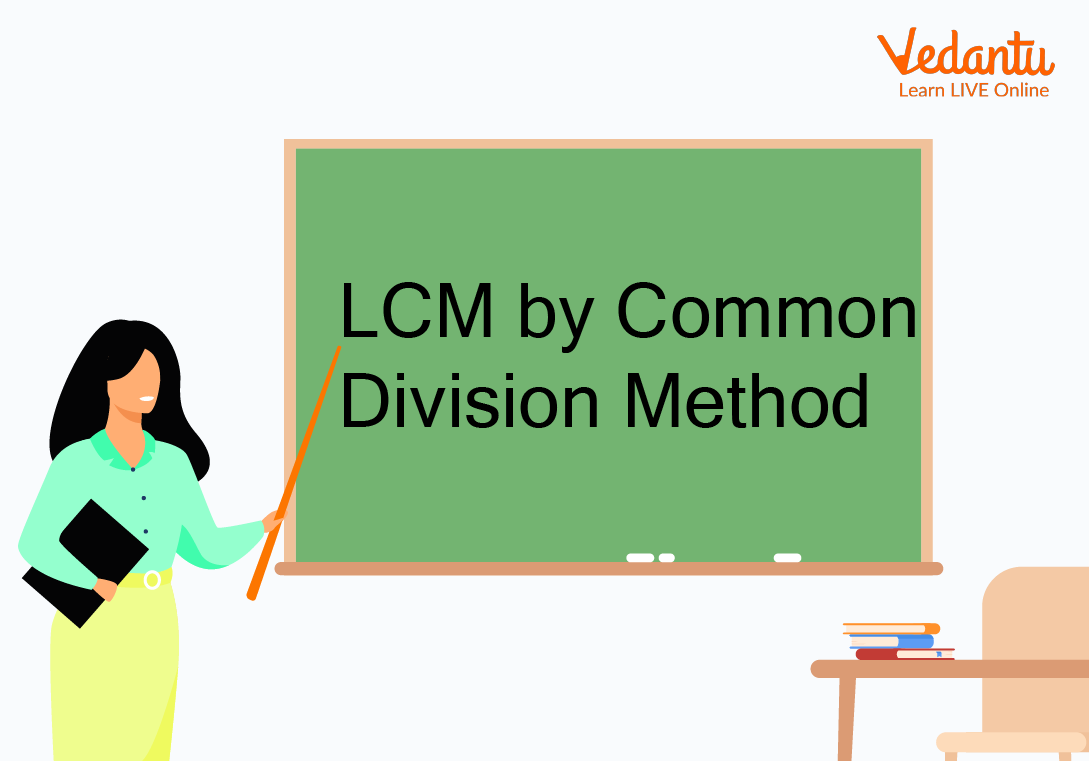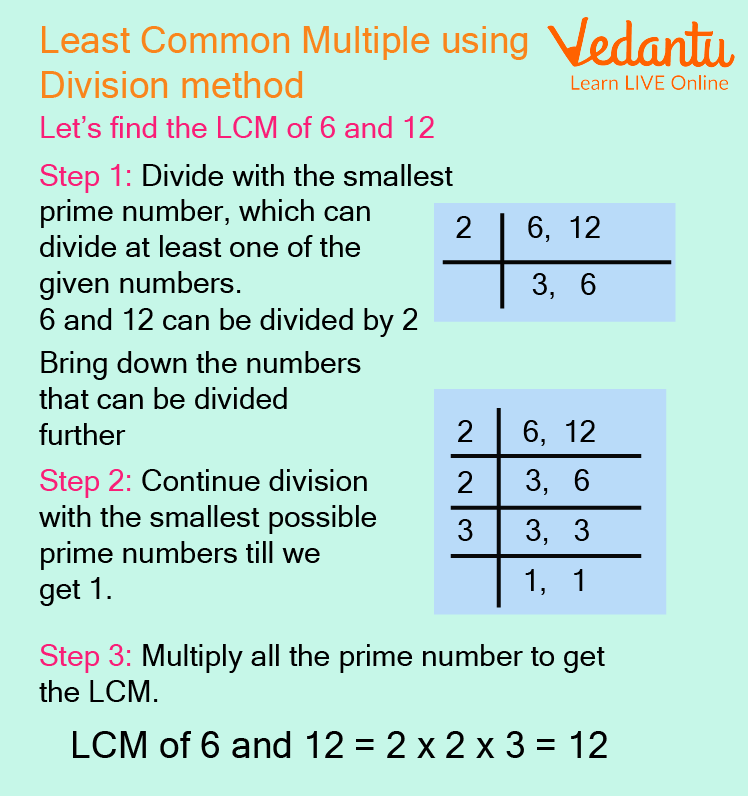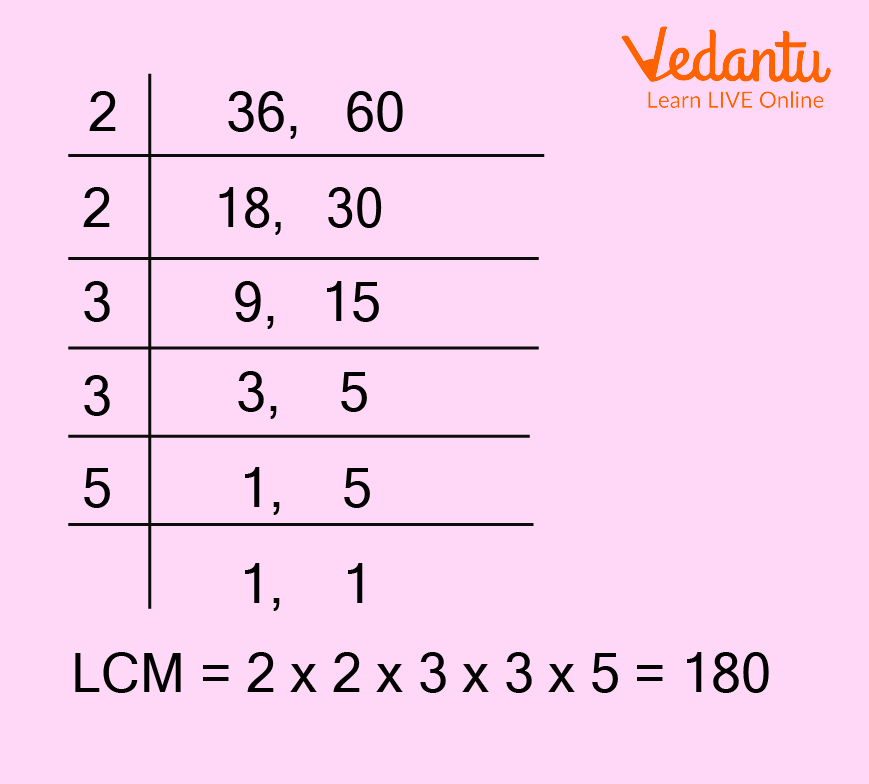Courses
Courses for Kids
Free study material
Free LIVE classes
More

# What is LCM, and How to Take LCM?LIVE
Join Vedantu’s FREE Mastercalss

## What is LCM?

We first need to understand what is LCM (Least common multiple)? Why is it used? It is the lowest number that is completely divisible by each of the given numbers. LCM stands for "Least Common Multiple" which is the smallest positive integer that is a multiple of two or more integers added together. We can find the L.C.M of two or more numbers using three methods. In this article, we will dive into the basics of the LCM method and understand how to find LCM and represent it.Full Form of LCM

## How to Find LCM by Listing Method

We can find out the common multiples of two or more numbers. Out of these repeated multiples, the LCM of two numbers can be calculated.

Steps to calculate LCM by listing method:

• List the initial range of each number's multiples.

• Search for multiples that appear on all number lists. Write out additional multiples for each number if there aren't any common multiples in the lists.

• Find the least quantity that appears on both lists.

• This is the LCM number.

Let’s take the LCM method example for the above method:

Example: Find the LCM of numbers 4 and 5 by listing methods.

Ans: LCM of 4 and 5 by the listing method:

Multiples of 4: 4, 8, 12, 16, 20, 24, 28, 32, 36, 40, ..

Multiples of 5: 5, 10, 15, 20, 25, 30, 35, 40, …

So Common Multiples are: 20, 40, …

according to the least method, the LCM of 4 and 5 is 20.

## How to Find LCM by Prime Factorisation Method

Using the prime factorization method, we can find out the prime factors of the numbers.

Steps to be followed to calculate the LCM by the prime factors method is:

• Step 1: Finding each number's prime factorization is the first step in computing the LCM using the prime factors approach.

• Step 2: When you write each number as a product of primes.

• Step 3: Now take the highest power of each prime number.

• Step 4: To obtain the LCM, multiply the prime numbers.

Let’s take the LCM method example for the above method:

Example: Calculate the LCM of 50 and 100.

Ans: Steps to be followed to calculate LCM are:

• Finding each number's prime factorization:

$50: 2 \times 5 \times 5$

$100: 2 \times 2 \times 5 \times 5$

• When you write each number as a product of primes.

$50: 2 \times 5 \times 5$

$100: 2 \times 2 \times 5 \times 5$

• Now take the highest power of each prime number. Here the highest power of 2 and 5 is 2. Therefore,

$2 \times 2 \times 5 \times 5$

• To obtain the LCM, multiply the numbers

Multiplying $2 \times 2 \times 5 \times 5=100$

## How to Find LCM by Division MethodLCM by Standard Division Method

To calculate the LCM of two numbers using the division method, we have to follow the steps given below:

• Step 1: To find the LCM by division method, we write the given numbers in a row separately by commas, then divide the numbers by a common prime number. Find a prime number of a factor of at least one of the given numbers. Put this prime number to the left of the given numbers.

• Step 2: We stop dividing after reaching the prime numbers. The product of common and uncommon prime factors is the LCM of given numbers. (It means if the prime number in step 1 is a factor of the number, divide the number by the prime and write the quotient below. If the prime number in step 1 is not a factor of the number, write the number in the row below as it is. Continue the steps until only one is left in the last row.)

Let’s take the LCM method example for the above method:

Examples: Find the least common multiple (LCM) of 6 and 12 using the division method.

Ans: Step-by-step explanation to solve LCM by the common division method is given below:Step-by-Step Explanation to Solve LCM by Common Division Method

## Solved Examples

Q1. Find the least common multiple (LCM) of 36 and 60 using the division method.

Ans: Step-by-step explanation to solve LCM by the common division method is given below:LCM by Division Method

LCM of 36 and 60 is 2 ×2 × 3 × 3 × 5 = 180

Q2. What is the least common multiple of 980 and 9000 using the prime factorization method?

Ans: Steps to find LCM

Find the prime factorization of 980

980 = 2 × 2 × 5 × 7 × 7

Find the prime factorization of 9000

9000 = 2 × 2 × 2 × 3 × 3 × 5 × 5 × 5

Multiply each factor the greater number of times it occurs in steps i) or ii) above to find the LCM:

LCM = 2 × 2 × 2 × 3 × 3 × 5 × 5 × 5 × 7 × 7

LCM = 441000

## Practice Questions:

Q1. Find the LCM of 4, 8, and 16 by using the listing method.

Ans: 16

Q2. Find the LCM of 14 and 16.

Ans: 112

Q3. Find the LCM using the prime factors method: 60 and 72.

Ans: 360

Q4. Find the least common multiple (LCM) using the division method of: 9, 12 and 36

Ans: 36

## Summary

Learning about LCM can be helpful when trying to solve problems or puzzles. For example, if we have three numbers and want to find the smallest number that is a multiple of both of those numbers, we would use the LCM method. In this article, we learned about the Least Common Multiple (LCM) and how it can be used to simplify multiple problems. We also explored three methods for finding LCM, and explained how to solve it. Now that you have a better understanding of what LCM is and why it's important, continue learning more about this intriguing number in future articles!

Last updated date: 29th Sep 2023
Total views: 116.1k
Views today: 3.16k

## FAQs on What is LCM, and How to Take LCM?

1. How do you use LCM in everyday life?

LCM concept is important to solve problems related to racetracks, traffic lights, to predict when an event occurs again over a same period of time etc. For example: A, B and C start at the same time in the same direction to run around a circular stadium. A completes a round in 252 seconds, B in 308 seconds and c in 198 seconds, all starting at the same point. After what time will they be at the starting point? So, here by using the LCM we can easily find that A, B and C will again meet at the starting point in 2772 sec.

2. What is the most common and easy way to find the LCM?

As we know there are three ways to find the LCM which are listing method, prime factorization, and division. From these three methods, the division method is the most common, fastest, and easiest of these three methods. It can be used for both small and large numbers.

3. How do you find the LCM of three numbers?

For each given number, determine all of its prime factors. List every prime number that was discovered, in the order that they appear for each given number. To get the LCM, multiply the highest powers of all prime factors together.